# What is Normal Distribution?

The normal distribution aka Gaussian distribution, is a continuous probability distribution that is described by Normal Equation given below:

# Normal Distribution — Why is it considered important?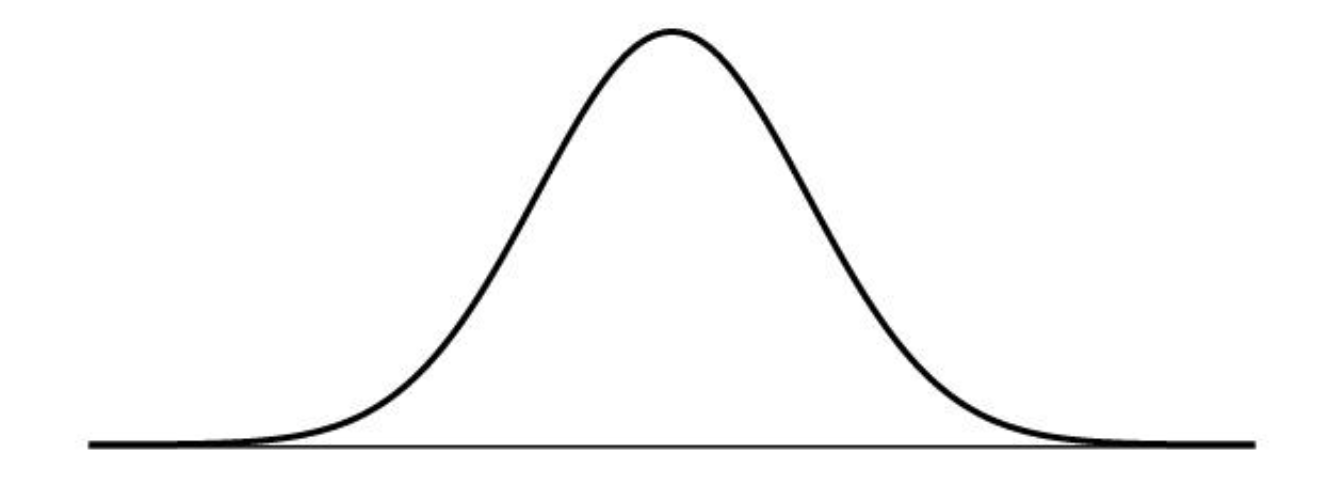Normal Distribution — The Bell Curve — The Gaussian Distribution

# Parameters of Normal Distribution

Normal distributions are defined by two parameters, the mean (μ) and the standard deviation (σ).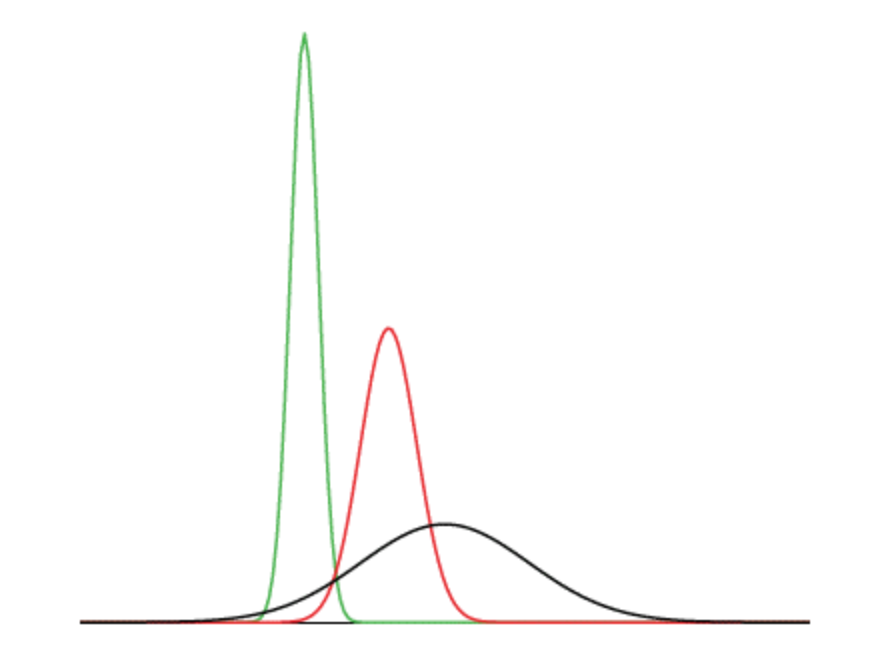Normal Distributions differing in Mean and Standard Deviations

## Mean

It determines the location of the peak, and most of the data points are clustered around the mean in a normal distribution graph.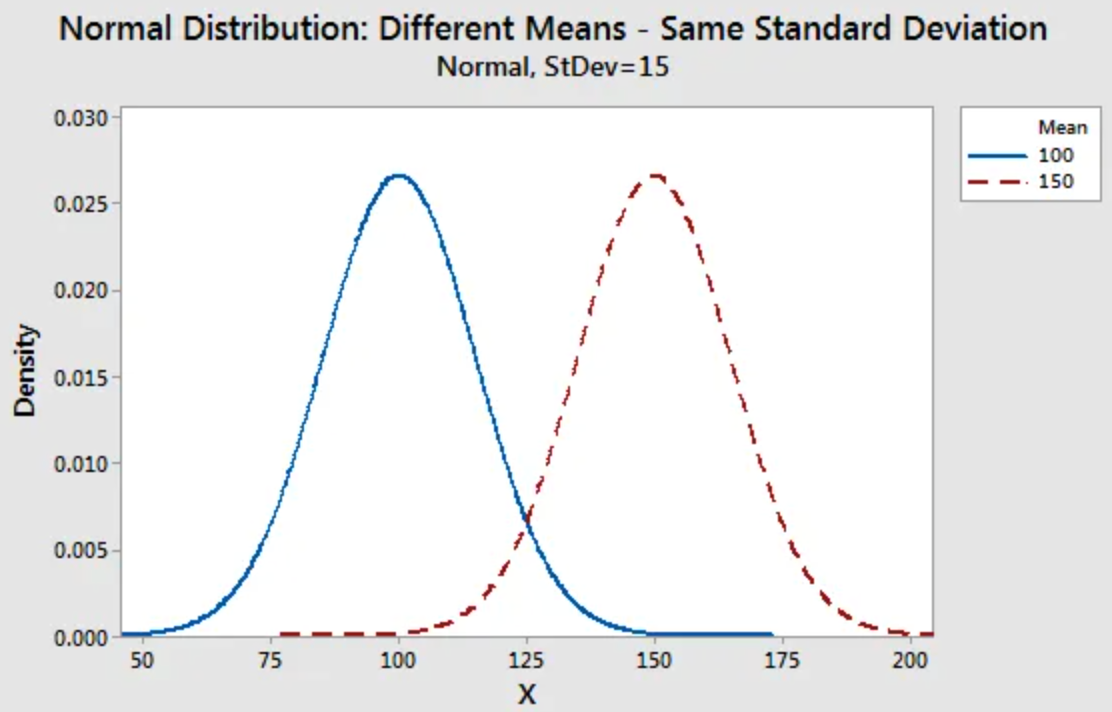Diagram shows how changing the mean shifts the entire curve left or right on the X-axis.

## Standard Deviation

It determines how far the data points are away from the mean and represents the distance between the mean and the data points.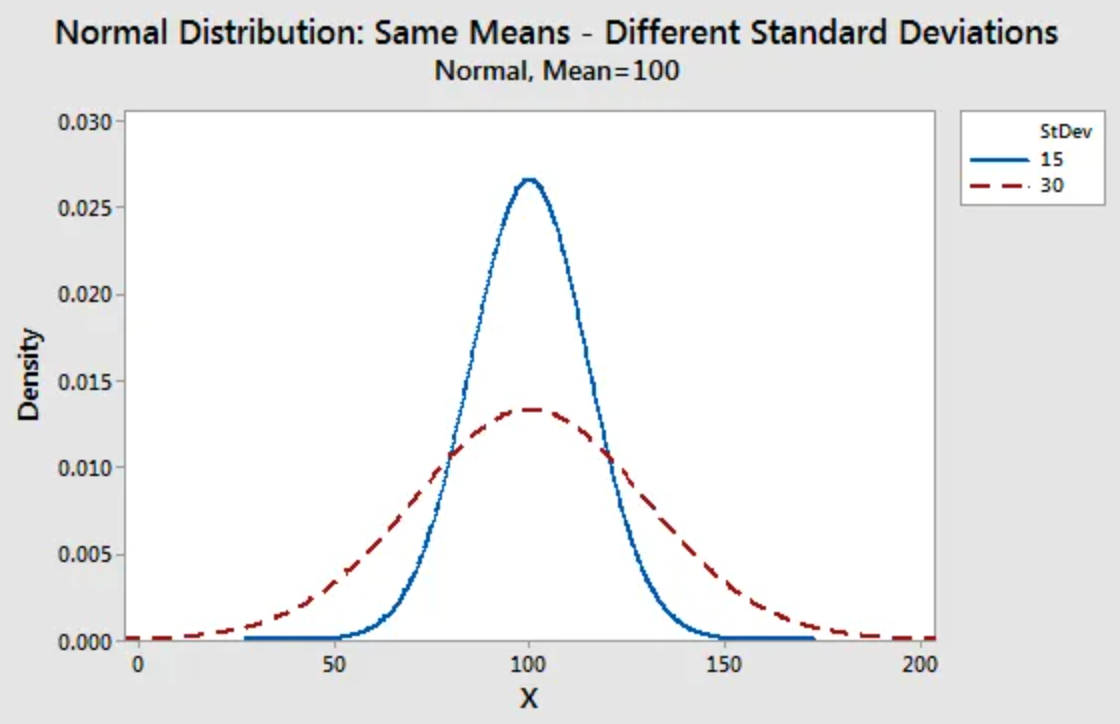Diagram shows how changing the standard deviation either tightens or spreads out the width of the distribution along the X-axis

# Properties of Normal Distribution

1. Mean, Median, and Mode are equal.
2. The curve is symmetric, with half of the values on the left and half of the values on the right.
3. The area under the curve is 1.
4. It has Zero Skewness and Kurtosis is equal to 3 (Mesokurtic)
5. A Normal distribution follows the Empirical rule, which states that:
• 95% of the data falls within 2 standard deviations of the mean
• 99.7% of the data falls within 3 standard deviations of the mean.

# Standard Scores

A value on the standard normal distribution is known as a Standard Score or a Z-score.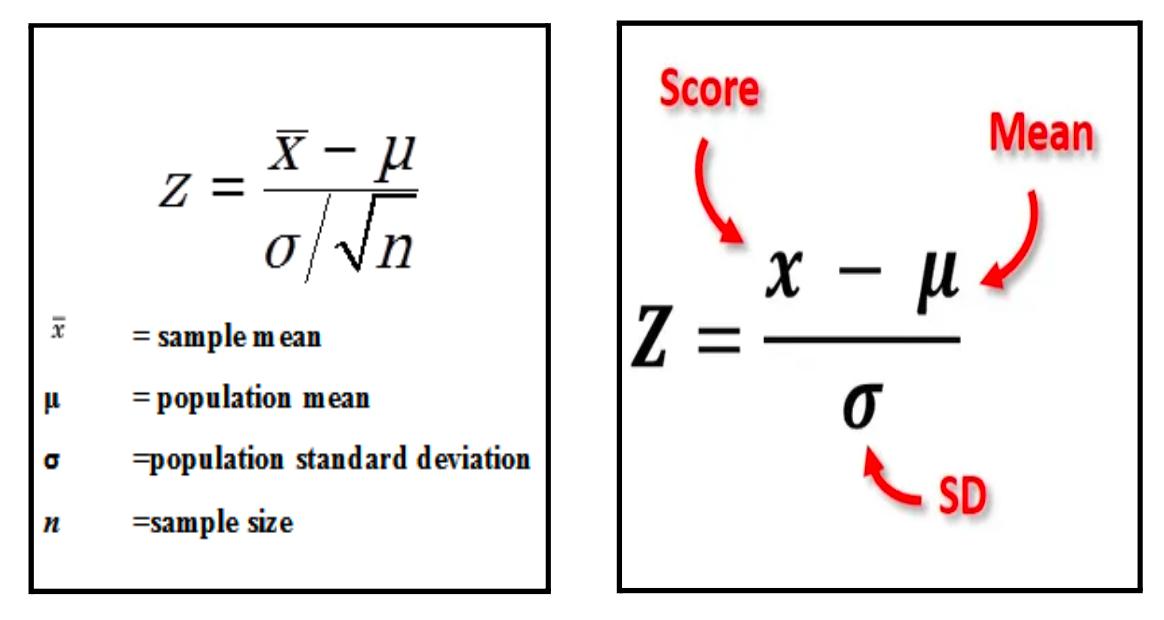Z score for Sample and Population

# Applications

Problem Statement:Let’s have data of heights of Indian men aged 18 to 24, which is approximately normally distributed with a mean of 65.5 inches and a standard deviation of 2.5 inches.